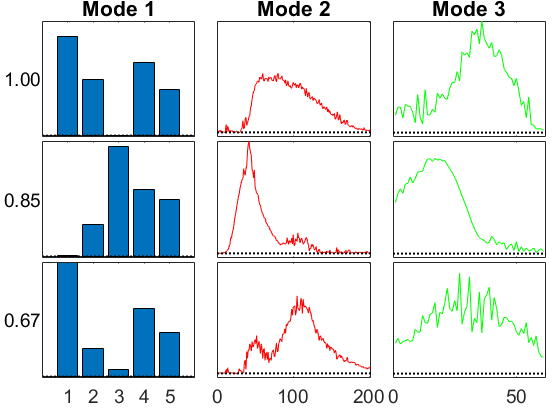# GCP-OPT Examples with Amino Acids Dataset

## Setup

We use the well known amino acids dataset for some tests. This data has some negative values, but the factorization itself should be nonnegative.

```rng('default'); %<- Setting random seed for reproducibility of this script

clear M fit

vizopts = {'PlotCommands',{@bar,@(x,y) plot(x,y,'r'),@(x,y) plot(x,y,'g')},...
'BottomSpace',0.1, 'HorzSpace', 0.04, 'Normalize', @(x) normalize(x,'sort',2)};
```

## CP-ALS

Just a reminder of what CP-ALS does.

```cnt = 1;

tic, M{cnt} = cp_als(X,3,'printitn',10); toc

fit(cnt) = 1 - norm(full(M{cnt})-X)/norm(X);
fprintf('Fit: %g\n', fit(cnt));

viz(M{cnt},'Figure',cnt,vizopts{:});
```
```CP_ALS:
Iter 10: f = 9.072207e-01 f-delta = 5.0e-02
Iter 20: f = 9.713716e-01 f-delta = 6.0e-04
Iter 30: f = 9.742235e-01 f-delta = 1.3e-04
Iter 32: f = 9.744253e-01 f-delta = 9.3e-05
Final f = 9.744253e-01
Elapsed time is 0.111475 seconds.
Fit: 0.974425
```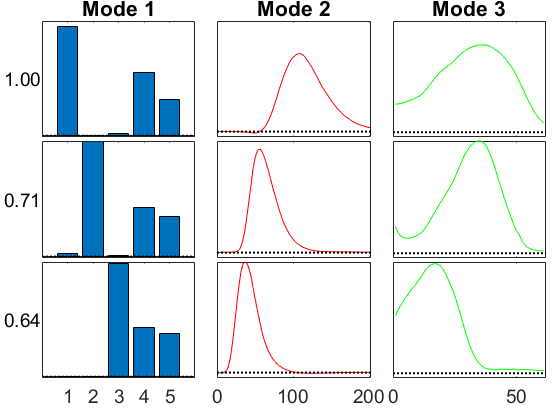## GCP with Gaussian

We can instead call the GCP with the Gaussian function.

```cnt = 2;
M{cnt} = gcp_opt(X,3,'type','Gaussian','printitn',10);

fit(cnt) = 1 - norm(full(M{cnt})-X)/norm(X);
fprintf('Fit: %g\n', fit(cnt));

viz(M{cnt},'Figure',cnt,vizopts{:});
```
```GCP-OPT-LBFGSB (Generalized CP Tensor Decomposition)

Tensor size: 5 x 201 x 61 (61305 total entries)
Generalized function Type: Gaussian
Objective function: @(x,m)(m-x).^2
Lower bound of factor matrices: -Inf
Optimization method: lbfgsb
Max iterations: 1000

Begin Main loop
Iter    10, f(x) = 1.606769e+08, ||grad||_infty = 4.59e+06
Iter    20, f(x) = 1.688031e+06, ||grad||_infty = 1.64e+05
Iter    30, f(x) = 1.448515e+06, ||grad||_infty = 1.75e+04
Iter    40, f(x) = 1.445322e+06, ||grad||_infty = 3.71e+03
Iter    50, f(x) = 1.445120e+06, ||grad||_infty = 1.88e+03
Iter    60, f(x) = 1.445110e+06, ||grad||_infty = 1.18e+02
Iter    66, f(x) = 1.445110e+06, ||grad||_infty = 2.15e+01
End Main Loop

Final objective: 1.4451e+06
Setup time: 0.05 seconds
Main loop time: 0.21 seconds
Outer iterations: 66
Total iterations: 142
L-BFGS-B Exit message: CONVERGENCE: REL_REDUCTION_OF_F_<=_FACTR*EPSMCH.
Fit: 0.974951
```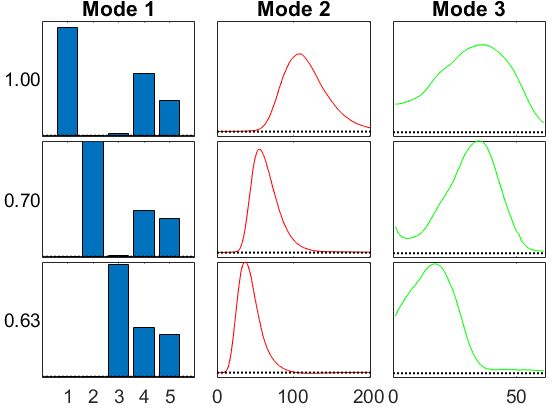## GCP with Gaussian and Missing Data

What if some data is missing?

```cnt = 3;

% Proportion of missing data
p = 0.35;

% Create a mask with the missing entries set to 0 and everything else 1
W = tensor(double(rand(size(X))>p));

% Fit the model, using the 'mask' option

fit(cnt) = 1 - norm(full(M{cnt})-X)/norm(X);
fprintf('Fit: %g\n', fit(cnt));

viz(M{cnt},'Figure',cnt,vizopts{:});
```
```GCP-OPT-LBFGSB (Generalized CP Tensor Decomposition)

Tensor size: 5 x 201 x 61 (61305 total entries)
Missing entries: 21604 (35%)
Generalized function Type: Gaussian
Objective function: @(x,m)(m-x).^2
Lower bound of factor matrices: -Inf
Optimization method: lbfgsb
Max iterations: 1000

Begin Main loop
Iter    10, f(x) = 1.218345e+07, ||grad||_infty = 1.11e+06
Iter    20, f(x) = 1.215698e+06, ||grad||_infty = 9.32e+04
Iter    30, f(x) = 9.243577e+05, ||grad||_infty = 5.99e+03
Iter    40, f(x) = 9.236897e+05, ||grad||_infty = 7.31e+02
Iter    50, f(x) = 9.236578e+05, ||grad||_infty = 1.62e+02
Iter    60, f(x) = 9.236571e+05, ||grad||_infty = 1.14e+02
Iter    62, f(x) = 9.236571e+05, ||grad||_infty = 1.34e+01
End Main Loop

Final objective: 9.2366e+05
Setup time: 0.01 seconds
Main loop time: 0.20 seconds
Outer iterations: 62
Total iterations: 133
L-BFGS-B Exit message: CONVERGENCE: REL_REDUCTION_OF_F_<=_FACTR*EPSMCH.
Fit: 0.974844
```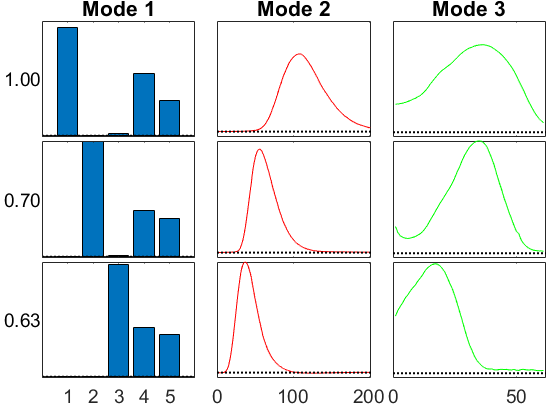We can also use stochastic gradient, though it's pretty slow for such a small tensor.

```cnt = 4;

fit(cnt) = 1 - norm(full(M{cnt})-X)/norm(X);
fprintf('Fit: %g\n', fit(cnt));

viz(M{cnt},'Figure',cnt,vizopts{:});
```
```GCP-OPT-ADAM (Generalized CP Tensor Decomposition)

Tensor size: 5 x 201 x 61 (61305 total entries)
Generalized function Type: Gaussian
Objective function: @(x,m)(m-x).^2
Lower bound of factor matrices: -Inf
Max iterations (epochs): 1000
Iterations per epoch: 1000
Learning rate / decay / maxfails: 0.001 0.1 1
Function Sampler: uniform with 5000 samples
Gradient Sampler: uniform with 500 samples

Begin Main loop
Initial f-est: 2.376253e+09
Epoch  1: f-est = 1.562517e+09, step = 0.001
Epoch  2: f-est = 1.184782e+09, step = 0.001
Epoch  3: f-est = 9.455418e+08, step = 0.001
Epoch  4: f-est = 7.906016e+08, step = 0.001
Epoch  5: f-est = 6.720333e+08, step = 0.001
Epoch  6: f-est = 5.629400e+08, step = 0.001
Epoch  7: f-est = 4.587569e+08, step = 0.001
Epoch  8: f-est = 3.660608e+08, step = 0.001
Epoch  9: f-est = 2.845412e+08, step = 0.001
Epoch 10: f-est = 2.102683e+08, step = 0.001
Epoch 11: f-est = 1.430214e+08, step = 0.001
Epoch 12: f-est = 8.715441e+07, step = 0.001
Epoch 13: f-est = 4.704066e+07, step = 0.001
Epoch 14: f-est = 2.347157e+07, step = 0.001
Epoch 15: f-est = 1.150061e+07, step = 0.001
Epoch 16: f-est = 6.137558e+06, step = 0.001
Epoch 17: f-est = 4.016841e+06, step = 0.001
Epoch 18: f-est = 3.055556e+06, step = 0.001
Epoch 19: f-est = 2.555754e+06, step = 0.001
Epoch 20: f-est = 2.198882e+06, step = 0.001
Epoch 21: f-est = 1.909576e+06, step = 0.001
Epoch 22: f-est = 1.725434e+06, step = 0.001
Epoch 23: f-est = 1.634091e+06, step = 0.001
Epoch 24: f-est = 1.572912e+06, step = 0.001
Epoch 25: f-est = 1.558587e+06, step = 0.001
Epoch 26: f-est = 1.547261e+06, step = 0.001
Epoch 27: f-est = 1.540582e+06, step = 0.001
Epoch 28: f-est = 1.553539e+06, step = 0.001, nfails = 1 (resetting to solution from last epoch)
Epoch 29: f-est = 1.533630e+06, step = 0.0001
Epoch 30: f-est = 1.534586e+06, step = 0.0001, nfails = 2 (resetting to solution from last epoch)
End Main Loop

Final f-est: 1.5336e+06
Setup time: 0.03 seconds
Main loop time: 25.05 seconds
Total iterations: 30000
Fit: 0.974923
```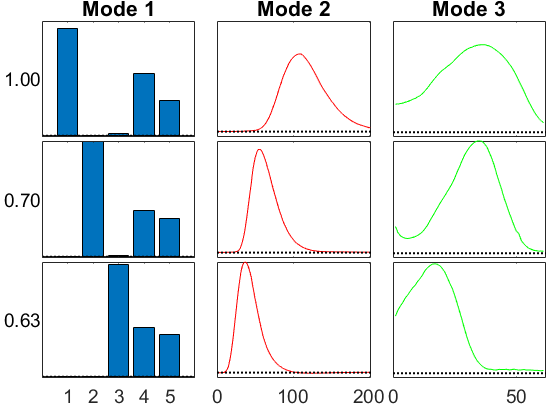## GCP with Gamma (terrible!)

We can try Gamma, but it's not really the right distribution and produces a terrible result.

```cnt = 5;

Y = tensor(X(:) .* (X(:) > 0), size(X));
M{cnt} = gcp_opt(Y,3,'type','Gamma','printitn',25);

fit(cnt) = 1 - norm(full(M{cnt})-X)/norm(X);
fprintf('Fit: %g\n', fit(cnt));

viz(M{cnt},'Figure',cnt,vizopts{:});
```
```Warning: Using 'Gamma' type but tensor X
is not nonnegative

GCP-OPT-LBFGSB (Generalized CP Tensor Decomposition)

Tensor size: 5 x 201 x 61 (61305 total entries)
Generalized function Type: Gamma
Objective function: @(x,m)x./(m+1e-10)+log(m+1e-10)
Lower bound of factor matrices: 0
Optimization method: lbfgsb
Max iterations: 1000

Begin Main loop
Iter    25, f(x) = 3.083247e+05, ||grad||_infty = 2.12e+03
Iter    50, f(x) = 3.061446e+05, ||grad||_infty = 1.57e+03
Iter    75, f(x) = 3.042764e+05, ||grad||_infty = 3.33e+03
Iter   100, f(x) = 3.030117e+05, ||grad||_infty = 2.58e+03
Iter   125, f(x) = 3.020461e+05, ||grad||_infty = 2.82e+03
Iter   150, f(x) = 3.013471e+05, ||grad||_infty = 2.13e+03
Iter   175, f(x) = 2.999417e+05, ||grad||_infty = 7.65e+03
Iter   200, f(x) = 2.978982e+05, ||grad||_infty = 2.87e+04
Iter   225, f(x) = 2.963939e+05, ||grad||_infty = 5.74e+03
Iter   250, f(x) = 2.954331e+05, ||grad||_infty = 9.36e+03
Iter   275, f(x) = 2.943538e+05, ||grad||_infty = 3.05e+03
Iter   300, f(x) = 2.931734e+05, ||grad||_infty = 3.03e+03
Iter   325, f(x) = 2.924773e+05, ||grad||_infty = 3.29e+03
Iter   350, f(x) = 2.916153e+05, ||grad||_infty = 2.73e+03
Iter   375, f(x) = 2.906693e+05, ||grad||_infty = 1.14e+04
Iter   400, f(x) = 2.894365e+05, ||grad||_infty = 2.82e+03
Iter   425, f(x) = 2.886059e+05, ||grad||_infty = 3.85e+03
Iter   450, f(x) = 2.878322e+05, ||grad||_infty = 3.86e+03
Iter   475, f(x) = 2.871707e+05, ||grad||_infty = 8.92e+03
Iter   500, f(x) = 2.868003e+05, ||grad||_infty = 8.36e+03
Iter   525, f(x) = 2.863173e+05, ||grad||_infty = 6.77e+03
Iter   550, f(x) = 2.857842e+05, ||grad||_infty = 5.46e+03
Iter   575, f(x) = 2.852310e+05, ||grad||_infty = 4.58e+03
Iter   600, f(x) = 2.847018e+05, ||grad||_infty = 2.46e+03
Iter   625, f(x) = 2.844237e+05, ||grad||_infty = 2.23e+03
Iter   650, f(x) = 2.840324e+05, ||grad||_infty = 2.68e+03
Iter   675, f(x) = 2.831906e+05, ||grad||_infty = 6.25e+03
Iter   700, f(x) = 2.815585e+05, ||grad||_infty = 3.00e+03
Iter   725, f(x) = 2.806378e+05, ||grad||_infty = 3.05e+03
Iter   750, f(x) = 2.800926e+05, ||grad||_infty = 2.42e+03
Iter   775, f(x) = 2.798322e+05, ||grad||_infty = 2.42e+03
Iter   800, f(x) = 2.795942e+05, ||grad||_infty = 2.36e+03
Iter   825, f(x) = 2.793289e+05, ||grad||_infty = 2.59e+03
Iter   850, f(x) = 2.791985e+05, ||grad||_infty = 2.53e+03
Iter   875, f(x) = 2.789471e+05, ||grad||_infty = 3.60e+03
Iter   900, f(x) = 2.785491e+05, ||grad||_infty = 3.91e+03
Iter   925, f(x) = 2.781030e+05, ||grad||_infty = 4.35e+03
Iter   950, f(x) = 2.776572e+05, ||grad||_infty = 1.69e+03
Iter   975, f(x) = 2.773212e+05, ||grad||_infty = 3.80e+03
Iter  1000, f(x) = 2.769849e+05, ||grad||_infty = 1.81e+03
End Main Loop

Final objective: 2.7698e+05
Setup time: 0.02 seconds
Main loop time: 3.52 seconds
Outer iterations: 1000
Total iterations: 2156
L-BFGS-B Exit message: UNRECOGNIZED EXIT FLAG
Fit: 0.403596
```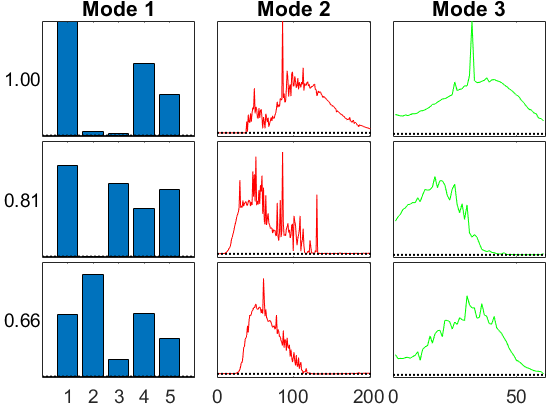## GCP with Huber + Lower Bound

Huber works well. By default, Huber has no lower bound. To add one, we have to pass in the func/grad/lower information explicitly. We can use gcp_fg_setup to get the func/grad parameters.

```cnt = 6;

% Call helper function tt_gcp_fg_setup to get the function and gradient handles
[fh,gh] = tt_gcp_fg_setup('Huber (0.25)');

fit(cnt) = 1 - norm(full(M{cnt})-X)/norm(X);
fprintf('Fit: %g\n', fit(cnt));

viz(M{cnt},'Figure',cnt,vizopts{:});
```
```GCP-OPT-LBFGSB (Generalized CP Tensor Decomposition)

Tensor size: 5 x 201 x 61 (61305 total entries)
Generalized function Type: user-specified
Objective function: @(x,m)(x-m).^2.*(abs(x-m)<0.25)+(0.5.*abs(x-m)-0.0625).*(abs(x-m)>=0.25)
Lower bound of factor matrices: 0
Optimization method: lbfgsb
Max iterations: 1000

Begin Main loop
Iter    25, f(x) = 4.493693e+05, ||grad||_infty = 9.33e+03
Iter    50, f(x) = 8.860955e+04, ||grad||_infty = 8.62e+03
Iter    75, f(x) = 8.304119e+04, ||grad||_infty = 3.40e+03
Iter    91, f(x) = 8.303986e+04, ||grad||_infty = 3.39e+03
End Main Loop

Final objective: 8.3040e+04
Setup time: 0.03 seconds
Main loop time: 0.21 seconds
Outer iterations: 91
Total iterations: 187
Fit: 0.973484
```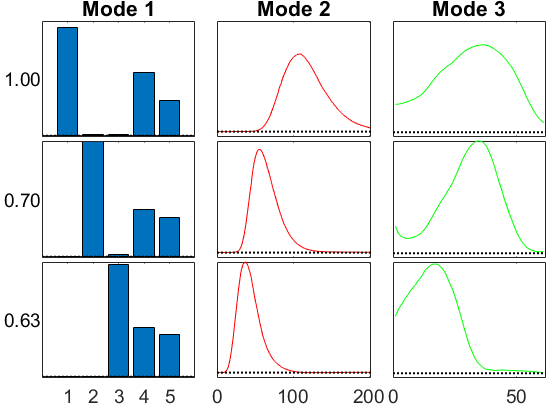## GCP with Beta

This is also pretty bad, which gives an idea of the struggle of choosing the wrong distribution. It can work a little bit, but it's clearly the wrong objective.

```cnt = 7;

M{cnt} = gcp_opt(X,3,'type','beta (0.75)','printitn',25);

fit(cnt) = 1 - norm(full(M{cnt})-X)/norm(X);
fprintf('Fit: %g\n', fit(cnt));
viz(M{cnt},'Figure',cnt,vizopts{:});
```
```Warning: Using 'beta' type but tensor X
is not nonnegative

GCP-OPT-LBFGSB (Generalized CP Tensor Decomposition)

Tensor size: 5 x 201 x 61 (61305 total entries)
Generalized function Type: beta (0.75)
Objective function: @(x,m)(1.33333).*(m+1e-10).^(0.75)-(-4).*x.*(m+1e-10).^(-0.25)
Lower bound of factor matrices: 0
Optimization method: lbfgsb
Max iterations: 1000

Begin Main loop
Positive dir derivative in projection
Using the backtracking step
Iter    25, f(x) = 9.861901e+06, ||grad||_infty = 9.24e+15
Iter    40, f(x) = 9.818012e+06, ||grad||_infty = 8.54e+15
End Main Loop

Final objective: 9.8180e+06
Setup time: 0.01 seconds
Main loop time: 1.21 seconds
Outer iterations: 40
Total iterations: 142
L-BFGS-B Exit message: CONVERGENCE: REL_REDUCTION_OF_F_<=_FACTR*EPSMCH.
Fit: 0.56936
```